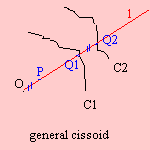# (generalized) cissoid

## main

last updated: 2003-12-09Given curves C1 and C2 and a fixed point O. Draw lines l through O that intersect C1 in Q1, and C2 in Q2. Then the cissoid is defined as the collection of points P (on l) for which OP is equal to the distance between the intersections (Q1Q2).

Some special cases of the cissoid:

 curve C1 curve C2 pole O cissoid line line parallel to C1 any point line circle center of the circle conchoid (of Nicomedes) circle concentric circle center circle line tangent to the circle on circle, opposite the tangent cissoid (of Diocles) line tangent to the circle on circle oblique cissoid radial line on circle (right) strophoid circle of equal size any point hippopede

The first cissoid to be discovered was the cissoid of Diocles.